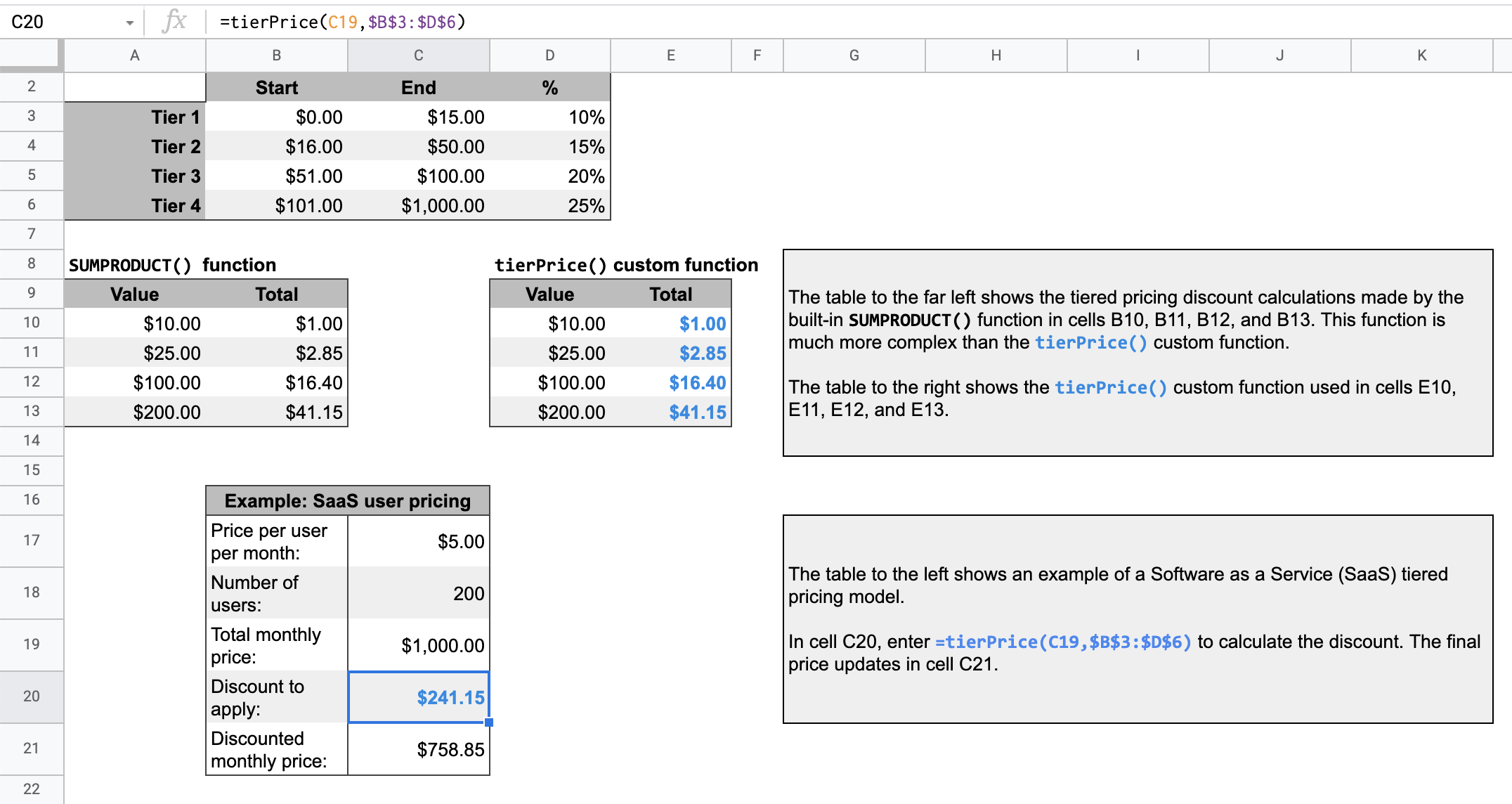# Calculate a tiered pricing discount

Stay organized with collections Save and categorize content based on your preferences.

Coding level: Beginner
Duration: 10 minutes
Project type: Custom function

## Objectives

• Understand what the solution does.
• Understand what the Apps Script services do within the solution.
• Set up the script.
• Run the script.

If you offer a tiered pricing system for your customers, this custom function makes it easier to calculate the discount amounts for your prices.

Though you could use the built-in function `SUMPRODUCT` to make a tiered pricing calculation, using `SUMPRODUCT` is more complex and less flexible than this solution's custom function.### How it works

A tiered pricing model means that the cost of goods or services goes down based on the quantity purchased.

For example, imagine you have two tiers, one that ranges from \$0-\$500 and is discounted by 10% and one that ranges from \$501-\$1,000 and is discounted by 20%. If the total price you need to calculate a discount for is \$700, the script multiplies the first \$500 by 10% and the remaining \$200 by 20%, for a total discount of \$90.

For a given total price, the script loops through the specified tiers in the tier pricing table. For each portion of the total price that falls within a tier, that portion is multiplied by the tier's associated percent value. The result is the sum of each tier's calculation.

### Apps Script services

This solution uses the following service:

• Spreadsheet service–Takes the given value and calculates what portion of the value to multiply by each tier's percent discount.

## Prerequisites

To use this sample, you need the following prerequisites:

## Set up the script

Click the button below to make a copy of the Tier pricing custom function spreadsheet. The Apps Script project for this solution is attached to the spreadsheet.

## Run the script

1. In your copied spreadsheet, the table at row 16 shows a sample price calculation for a Software as a Service (SaaS) product.
2. To calculate the discount amount, in cell `C20`, enter `=tierPrice(C19,\$B\$3:\$D\$6)`. The final price updates in cell `C21`. If you're in a location that uses decimal commas, you might need to enter `=tierPrice(C19;\$B\$3:\$D\$6)` instead.

## Review the code

To review the Apps Script code for this solution, click View source code below:

### Code.gs

solutions/custom-functions/tier-pricing/Code.js
```// To learn how to use this script, refer to the documentation:

/*

you may not use this file except in compliance with the License.
You may obtain a copy of the License at

Unless required by applicable law or agreed to in writing, software
WITHOUT WARRANTIES OR CONDITIONS OF ANY KIND, either express or implied.
See the License for the specific language governing permissions and
*/

/**
* Calculates the tiered pricing discount.
*
* You must provide a value to calculate its discount. The value can be a string or a reference
* to a cell that contains a string.
* You must provide a data table range, for example, \$B\$4:\$D\$7, that includes the
* tier start, end, and percent columns. If your table has headers, don't include
* the headers in the range.
*
* @param {string} value The value to calculate the discount for, which can be a string or a
* reference to a cell that contains a string.
* @param {string} table The tier table data range using A1 notation.
* @return number The total discount amount for the value.
* @customfunction
*
*/
function tierPrice(value, table) {
let total = 0;
// Creates an array for each row of the table and loops through each array.
for (let [start, end, percent] of table) {
// Checks if the value is less than the starting value of the tier. If it is less, the loop stops.
if (value < start) {
break;
}
// Calculates the portion of the value to be multiplied by the tier's percent value.
let amount = Math.min(value, end) - start;
// Multiplies the amount by the tier's percent value and adds the product to the total.
total += amount * percent;
}
}
```

## Modifications

You can edit the custom function as much as you'd like to fit your needs. Below is an optional addition to manually refresh custom function results.

#### Refresh cached results

Unlike built-in functions, Google caches custom functions to optimize performance. Therefore, if you change something within your custom function, such as a value that's being calculated, it might not immediately force an update. To refresh the function result manually, take the following steps:

1. Add a checkbox to an empty cell by clicking Insert > Checkbox.
2. Add the cell that has the checkbox as an extra parameter of the custom function. For example, if you add a checkbox to cell `D20`, update the `tierPrice()` function in cell `C20` to `=tierPrice(C19,\$B\$3:\$D\$6,D20)`.
3. Check or uncheck the checkbox to refresh the custom function results.

## Contributors

This sample is maintained by Google with the help of Google Developer Experts.

## Next steps

[{ "type": "thumb-down", "id": "missingTheInformationINeed", "label":"Missing the information I need" },{ "type": "thumb-down", "id": "tooComplicatedTooManySteps", "label":"Too complicated / too many steps" },{ "type": "thumb-down", "id": "outOfDate", "label":"Out of date" },{ "type": "thumb-down", "id": "samplesCodeIssue", "label":"Samples / code issue" },{ "type": "thumb-down", "id": "otherDown", "label":"Other" }]
[{ "type": "thumb-up", "id": "easyToUnderstand", "label":"Easy to understand" },{ "type": "thumb-up", "id": "solvedMyProblem", "label":"Solved my problem" },{ "type": "thumb-up", "id": "otherUp", "label":"Other" }]